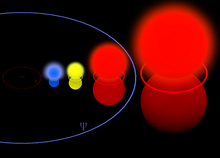Solar mass

﻿
Solar massSize and mass of very large stars: Most massive example, the blue Pistol Star (150$\begin{smallmatrix}M_\odot\end{smallmatrix}$). Others are Rho Cassiopeiae (40$\begin{smallmatrix}M_\odot\end{smallmatrix}$), Betelgeuse (20$\begin{smallmatrix}M_\odot\end{smallmatrix}$), and VY Canis Majoris (30-40$\begin{smallmatrix}M_\odot\end{smallmatrix}$). (The Sun (1$\begin{smallmatrix}M_\odot\end{smallmatrix}$) which is not visible in this thumbnail is included to illustrate the scale of example stars)

The solar mass ($\begin{smallmatrix}M_\odot\end{smallmatrix}$), 1.98892×1030 kg, is a standard unit of mass in astronomy, used to indicate the masses of other stars and galaxies. It is equal to the mass of the Sun, about two nonillion kilograms or about 332,950 times the mass of the Earth or 1,048 times the mass of Jupiter.$M_{\odot}=( 1.98892\ \pm\ 0.00025 )\ \times10^{30}\hbox{ kg}$

The solar mass can be determined from the length of the year, the distance of the Earth to the Sun (the astronomical unit) (AU), and the gravitational constant (G) as$M_\odot=\frac{4 \pi^2 \times (1{\rm AU})^3}{G\times(1{\rm year})^2}$.

The value of the gravitational constant was derived from 1798 measurements by Henry Cavendish using a torsion balance. The value obtained differed only by about 1% from the modern value. The diurnal parallax of the Sun was accurately measured during the transits of Venus in 1761 and 1769, yielding a value of 9″ (compared to the present 1976 value of 8.794148″). When the value of the diurnal parallax is known, the distance to the Sun can be determined from the geometry of the Earth.

The first person to estimate the mass of the Sun was Isaac Newton. In his work Principia, he estimated that the ratio of the mass of the Earth to the Sun was about 1/28,700. Later he determined that this value was based upon a faulty value for the solar parallax, which was used to estimate the distance to the Sun (1 AU). He revised his result to obtain a ratio of 1/169,282 in the third edition of the Principia. The current value for the solar parallax is smaller still, giving a mass ratio of 1/332,946.

As a unit of measurement, the solar mass came into use before the AU and the gravitational constant were precisely measured. This is because the determination of the relative mass of another planet in the Solar System or of a binary star in units of solar masses does not depend on these poorly known constants. So it was useful to express these masses in units of solar masses (see Gaussian gravitational constant).

The mass of the Sun changes slowly, compared to the lifetime of the Sun. Mass is lost due to two main processes in nearly equal amounts. First, in the Sun's core hydrogen is converted into helium by nuclear fusion, in particular the pp chain. Thereby mass is converted to energy in correspondence to the mass–energy equivalence. This energy is eventually radiated away by the Sun. The second process is the solar wind, which is the ejection of mainly protons and electrons to outer space.

Related units

One Solar mass, M, can be converted to related units:

It is also frequently useful in general relativity to express mass in units of length or time.$M_\odot \frac{G}{c^2} \approx 1.48~\mathrm{km};\ \ M_\odot \frac{G}{c^3} \approx 4.93~ \mathrm{\mu s}$

Wikimedia Foundation. 2010.

Look at other dictionaries:

• solar mass — the unit, equivalent to the mass of the sun, in which the masses of stars and other celestial objects are given: a black hole of one million solar masses. * * * …   Universalium

• solar mass — noun a unit, equal to the mass of the sun, used to express the mass of other stars etc …   Wiktionary

• Solar Mass —   A term used for materials used to absorb and store solar energy …   Energy terms

• solar mass — noun Date: 1941 the mass of the sun used as a unit for the expression of the masses of other celestial objects and equal to about 2 × 1030 kilograms …   New Collegiate Dictionary

• solar mass — noun : the mass of the sun amounting to 2×1033 grams and being used as a unit for the expression of the masses of other stars, nebulas, and galaxies …   Useful english dictionary

• solar mass —    see Sun …   Dictionary of units of measurement

• Mass — This article is about the scientific concept. For the substance of which all physical objects consist, see Matter. For other uses, see Mass (disambiguation). Classical mechanics …   Wikipedia

• solar — adjective a) Of or pertaining to the sun; proceeding from the sun; as, the solar system; solar light; solar rays; solar influence. b) Measured by the progress or revolution of the sun in the ecliptic; as, the solar year. See Also: extrasolar,… …   Wiktionary

• Mass-to-light ratio — In astrophysics and physical cosmology the mass to light ratio, normally designated with the symbol Υ is the quotient between the total mass of a spatial volume (typically on the scales of a galaxy or a cluster) and its luminosity. These… …   Wikipedia

• Solar radius — In astronomy, the solar radius is a unit of length used to express the size of stars. It is equal to the current radius of the Sun. Its value is::1,R {odot} = 6.960 imes 10^8,hbox{m} = 0.004652,hbox{AU} (Astronomical unit).The solar radius is… …   Wikipedia